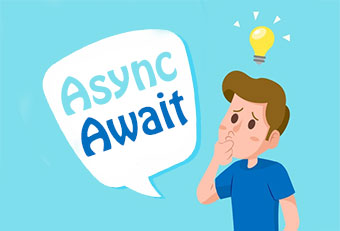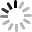# 簡單理解 JavaScript Async 和 Await## 什麼是 async？什麼是 await？

• 同步：在「同一個步道」比賽「接力賽跑」，當棒子沒有交給我，我就得等你，不能跑。
• 非同步：在「不 ( 非 ) 同步道」比賽「賽跑」，誰都不等誰，只要輪到我跑，我就開始跑。``````async function a(){
await b();
.....       // 等 b() 完成後才會執行
await c();
.....       // 等 c() 完成後才會執行
await new Promise(resolve=>{
.....
});
.....       // 上方的 promise 完成後才會執行
}
a();
a().then(()=>{
.....       // 等 a() 完成後接著執行
});
``````

## 利用 async 和 await 做個「漂亮的等待」

``````const delay = (s) => {
return new Promise(resolve => {
setTimeout(resolve,s);
});
};

delay().then(() => {
console.log(1);     // 顯示 1
return delay(1000); // 延遲ㄧ秒
}).then(() => {
console.log(2);     // 顯示 2
return delay(2000); // 延遲二秒
}).then(() => {
console.log(3);     // 顯示 3
});
``````

``````~async function{           // ~ 開頭表示直接執行這個 function，結尾有 ()
const delay = (s) => {
return new Promise(function(resolve){  // 回傳一個 promise
setTimeout(resolve,s);               // 等待多少秒之後 resolve()
});
};

console.log(1);      // 顯示 1
await delay(1000);   // 延遲ㄧ秒
console.log(2);      // 顯示 2
await delay(2000);   // 延遲二秒
console.log(3);      // 顯示 3
}();
``````

## 搭配 Promise

``````const count = (t,s) => {
let a = 0;
let timer = setInterval(() => {
console.log(`\${t}\${a}`);
a = a + 1;
if(a>5){
clearInterval(timer);
}
},s);
};

console.log(1);
count('haha', 100);
console.log(2);
````````````~async function(){
const count = (t,s) => {
return new Promise(resolve => {
let a = 0;
let timer = setInterval(() => {
console.log(`\${t}\${a}`);
a = a + 1;
if(a>5){
clearInterval(timer);
resolve();  // 表示完成
}
},s);
});
};

console.log(1);
await count('haha', 100);
console.log(2);
}();
````````````// HTML 為一個輸入框、一個按鈕和一個 h1 標籤
// <input id="a"></input><button id="b">send</button>
// <h1 id="h"></h1>

~async function(){
const input = () => {
return new Promise(resolve =>{
const btnClick = () =>{
h.insertAdjacentHTML('beforeend', a.value + '<br/>');   // 輸入後在 h1 裡添加內容
a.value = '';   // 清空輸入框
a.focus();      // 將焦點移至輸入框
b.removeEventListener('click', btnClick);  // removeEventListener 避免重複綁定事件
resolve();      // 完成
};
});
};
await input();     //  等待輸入，輸入後才會進行下一步
await input();
await input();
}();
``````## 搭配 Fetch

``````~async function(){
console.log('開始抓氣象');       // 先顯示「開始抓氣象」
await fetch('氣象局 json 網址')  // 帶有 await 的 fetch
.then(res => {
return res.json();
}).then(result => {
let city = result.cwbopendata.location.parameter.parameterValue;
let temp = result.cwbopendata.location.weatherElement.elementValue.value;
console.log(`\${city}的氣溫為 \${temp} 度 C`);
});
console.log('總算結束了');       // 抓完氣象之後再顯示「總算結束了」
}();
``````## 搭配 迴圈

``````let size = 30;
h.style.lineHeight = 0;
const timer = setInterval(()=>{
h.style.fontSize = size + 'px';
size = size + 1;
if(size >= 130){
clearInterval(timer);
}
},10);
``````

``````// HTML 為一個 h1 標籤 <h1 id="h">hello</h1>

~async function(){
const delay = t => {    // 先撰寫一個等待的 function
return new Promise(resolve => {
setTimeout(resolve, t);
});
};
h.style.linHeight = 0;
for(let i=30; i<130; i++){
h.style.fontSize = i + 'px';
await delay(10);    // 迴圈每次執行時，都會在這裡等待 10ms
}
}();
``````